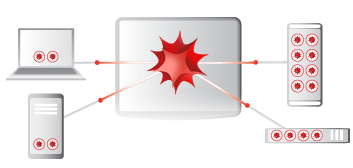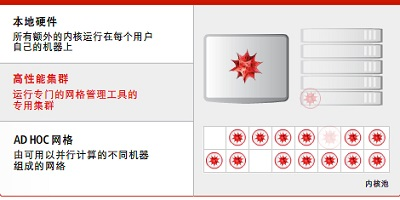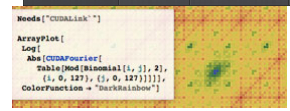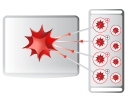成像光学 物理光学 光纤激光 照明 杂散光 二次开发 课程资讯 教学实验室 书籍中心 技术文章 常见问题 联系我们 工程顾问 光学成像设计 SYNOPSYS光学设计软件 ASAP光学设计软件 RP Fiber Power 平场聚焦透镜F-THETA SCAN LENS Mathematica全球现代技术计算终极系统 光学加工 PCGrate 光栅设计软件#### Wolfram gridMathematicaMathematica内含并行计算功能

gridMathematica 功能gridMathematica 作为一个集成的扩展系统可以使您的 Mathematica 许可证更加物有所值。每个 gridMathematica Server 赋予 Mathematica 用户一个由16个额外的能够在网络上使用的 Mathematica 计算内核组成的共享池，用以在多个CPU上运行分布式并行计算。

gridMathematica 提供：Mathematica 可以同时使用 gridMathematica 和一个 Mathematica Core Extension 吗？

gridMathematica 支持哪种网格管理系统？

gridMathematica 支持 Wolfram Lightweight Grid Manager、 Altair PBS Professional、Microsoft Windows Compute Cluster Server、Microsoft HPC Server、Platform LSF 和 Sun Grid Engine。另外，还可以查看支持平台页面。